# Resultant Force

## Key Stage 3

### Meaning

The Resultant Force is the overall force on an object.

A resultant force can be calculated by taking forces that act along the same line and add them together.
If the forces are in the same direction as each other, then they have a positive value.
If one force is acting in the opposite direction to another one of the forces is given a negative value.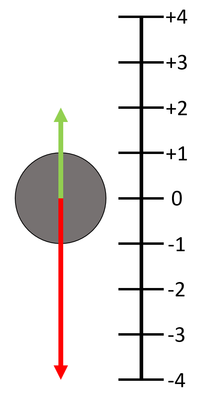If the 'up' direction is chosen to be positive then any vector pointing 'up' has a positive value and any vector pointing 'down' has a negative value. In this diagram the green vector has a value of +2 while the red vector has a value of -4. If the number lines represent the number of Newtons force then the Resultant Force is the two values added together. FR = (+2N) + (-4N) FR = -2N upwards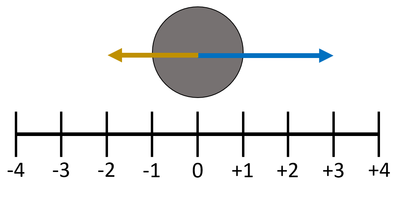If the 'right' direction is chosen to be positive then any vector pointing 'right' has a positive value and any vector pointing 'left' has a negative value. In this diagram the blue vector has a value of +3 while the yellow vector has a value of -2. If the number lines represent the number of Newtons force then the Resultant Force is the two values added together. FR = (+3N) + (-2N) FR = 1N to the right

### Examples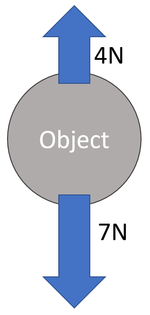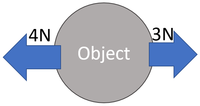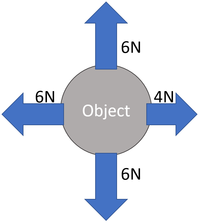Choose which directions are positive. Up is positive. Calculate the Resultant Force. There is 4N up and 7N down. FR = (+4N) + (-7N) FR = -3N up Choose which directions are positive. Right is positive. Calculate the Resultant Force. There is 4N left and 3N right. FR = (+3N) + (-4N) FR = -1N right Choose which directions are positive. Up is positive. Right is positive. Calculate the Resultant Force. There is 6N up and 6N down. FVertical = (+6N) + (-6N) FVertical = 0N up There is 6N left and 4N right. FHorizontal = (+4N) + (-6N) FHorizontal = -2N right

## Key Stage 4

### Meaning

The resultant force is the sum of all forces acting on an object.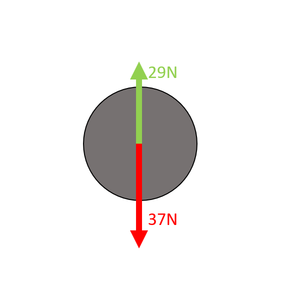Choose which directions are positive. Up is positive. Calculate the Resultant Force There is 29N up and 37N down. FR = (+29N) + (-37N) FR = -8N upwards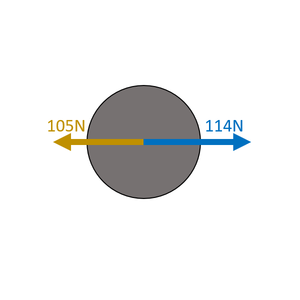Choose which directions are positive. Right is positive. Calculate the Resultant Force There is 114N right and 105N left. FR = (+114N) + (-105N) FR = 9N right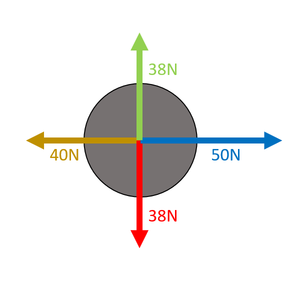Choose which directions are positive. Right is positive. Up is positive. Calculate the Resultant Force There is 38N up and 38N down. FR = (+38N) + (-38N) FR = 0N upwards Calculate the Resultant Force There is 50N right and 40N left. FR = (+50N) + (-40N) FR = 10N right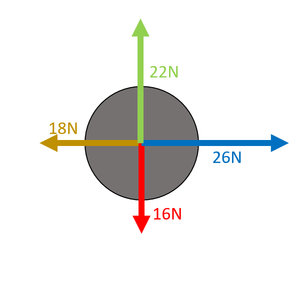Choose which directions are positive. Right is positive. Up is positive. Calculate the Resultant Force There is 22N up and 16N down. FVertical = (+22N) + (-16N) FVertical = 6N upwards Calculate the Resultant Force There is 26N right and 18N left. FHorizontal = (+26N) + (-18N) FHorizontal = 8N right Since there are two resultant forces that are perpendicular they form a right angle triangle and their hypotenuse is the magnitude of the resultant force. This can be found using Pythagoras' Theorem. $${F_R}^2 = {F_V}^2 + {F_H}^2$$ $$F_R = \sqrt{6^2 + 8^2}$$ $$F_R = \sqrt{100}$$ $$F_R = 10N$$ The angle from the horizontal can be found using Trigonometry. $$\tan \theta = \frac{Opposite}{Adjacent}$$ $$\tan \theta = \frac{6}{8}$$ $$\theta = 36.9^\circ$$ up from horizontal